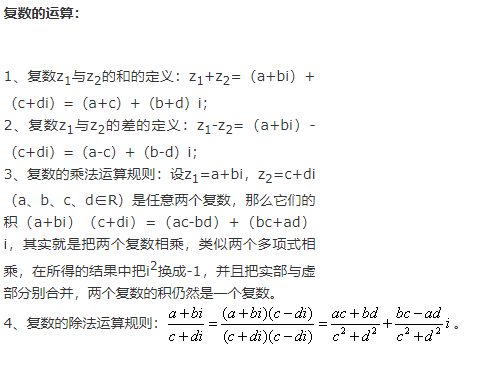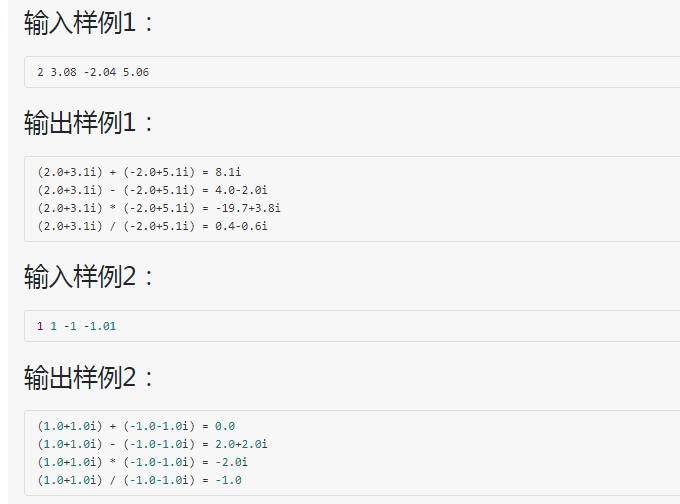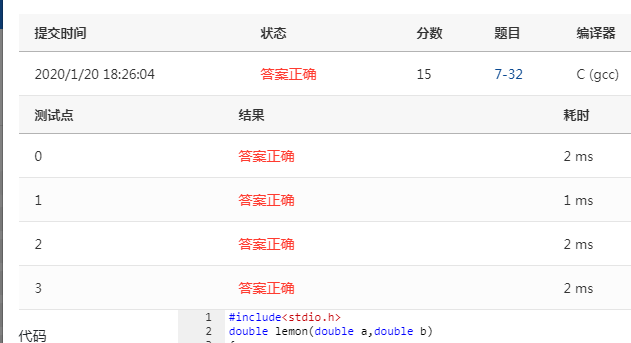• 7-36 复数四则运算 (15 分) 本要求编写程序，计算2个复数的和、差、积、商。 输入格式： 输入在一行中按照a1 b1 a2 b2的格式给出2个复数C1=a1+b1i和C2=a2+b2i的实部和虚部。题目保证C2不为0。 输出格式： ...
7-36 复数四则运算 (15 分)

本题要求编写程序，计算2个复数的和、差、积、商。

输入格式：

输入在一行中按照a1 b1 a2 b2的格式给出2个复数C1=a1+b1i和C2=a2+b2i的实部和虚部。题目保证C2不为0。

输出格式：

分别在4行中按照(a1+b1i) 运算符 (a2+b2i) = 结果的格式顺序输出2个复数的和、差、积、商，数字精确到小数点后1位。如果结果的实部或者虚部为0，则不输出。如果结果为0，则输出0.0。

输入样例1：

2 3.08 -2.04 5.06

输出样例1：

(2.0+3.1i) + (-2.0+5.1i) = 8.1i
(2.0+3.1i) - (-2.0+5.1i) = 4.0-2.0i
(2.0+3.1i) * (-2.0+5.1i) = -19.7+3.8i
(2.0+3.1i) / (-2.0+5.1i) = 0.4-0.6i

输入样例2：

1 1 -1 -1.01

输出样例2：

(1.0+1.0i) + (-1.0-1.0i) = 0.0
(1.0+1.0i) - (-1.0-1.0i) = 2.0+2.0i
(1.0+1.0i) * (-1.0-1.0i) = -2.0i
(1.0+1.0i) / (-1.0-1.0i) = -1.0

假如我们要对一个小数保留一个小数位而第二个小数位按照四舍五入的规则进行，
将数字扩大10倍，使得第四位小数称为新数字的第一位，然后我们可以给它加上0.5后再进行强制性转化。加0.5的原因就是：
假如这个小数位的数值大于0.5，加上0.5能够实现进位的作用，倘若小于0.5，就没有实现进位的作用。如此，就能实现小数位的四舍五入。然后我们再对这个数值
进行强制性转化，然后再处以10.0，在输出的时候用%0.1f以实现保留一位小数。#include<stdio.h>
double f(double a){
if(a<0){
return (int)(-a*10+0.5)/-10.0;
}else

return (int)(a*10+0.5)/10.0;
}
int main(){
double a1,b1,a2,b2,x,y,t;
scanf("%lf %lf %lf %lf",&a1,&b1,&a2,&b2);
//加
x=f(a1+a2);
y=f(b1+b2);
if(x==0&&y==0){   //printf("%+3d",a)  +表示输出的a正数时也带符号
printf("(%.1f%+.1fi) + (%.1f%+.1fi) = 0.0\n",f(a1),f(b1),f(a2),f(b2));
}else if(x==0){
printf("(%.1f%+.1fi) + (%.1f%+.1fi) = %.1fi\n",
f(a1),f(b1),f(a2),f(b2),y);
}else if(y==0){
printf("(%.1f%+.1fi) + (%.1f%+.1fi) = %.1f\n",
f(a1),f(b1),f(a2),f(b2),x);
}else{
printf("(%.1f%+.1fi) + (%.1f%+.1fi) = %.1f%+.1fi\n",
f(a1),f(b1),f(a2),f(b2),x,y);
}

//减
x=f(a1-a2);
y=f(b1-b2);
if(x==0&&y==0){
printf("(%.1f%+.1fi) - (%.1f%+.1fi) = 0.0\n",f(a1),f(b1),f(a2),f(b2));
}else if(x==0){
printf("(%.1f%+.1fi) - (%.1f%+.1fi) = %.1fi\n",
f(a1),f(b1),f(a2),f(b2),y);
}else if(y==0){
printf("(%.1f%+.1fi) - (%.1f%+.1fi) = %.1f\n",
f(a1),f(b1),f(a2),f(b2),x);
}else{
printf("(%.1f%+.1fi) - (%.1f%+.1fi) = %.1f%+.1fi\n",
f(a1),f(b1),f(a2),f(b2),x,y);
}
//乘
x=f(a1*a2-b1*b2);
y=f(b1*a2+a1*b2);

if(x==0&&y==0){
printf("(%.1f%+.1fi) * (%.1f%+.1fi) = 0.0\n",f(a1),f(b1),f(a2),f(b2));
}else if(x==0){
printf("(%.1f%+.1fi) * (%.1f%+.1fi) = %.1fi\n",
f(a1),f(b1),f(a2),f(b2),y);
}else if(y==0){
printf("(%.1f%+.1fi) * (%.1f%+.1fi) = %.1f\n",
f(a1),f(b1),f(a2),f(b2),x);
}else{
printf("(%.1f%+.1fi) * (%.1f%+.1fi) = %.1f%+.1fi\n",
f(a1),f(b1),f(a2),f(b2),x,y);
}
//除
t=a2*a2+b2*b2;
x=f((a1*a2+b1*b2)/t);
y=f((b1*a2-a1*b2)/t);
if(x==0&&y==0){
printf("(%.1f%+.1fi) / (%.1f%+.1fi) = 0.0\n",f(a1),f(b1),f(a2),f(b2));
}else if(x==0){
printf("(%.1f%+.1fi) / (%.1f%+.1fi) = %.1fi\n",
f(a1),f(b1),f(a2),f(b2),y);
}else if(y==0){
printf("(%.1f%+.1fi) / (%.1f%+.1fi) = %.1f\n",
f(a1),f(b1),f(a2),f(b2),x);
}else{
printf("(%.1f%+.1fi) / (%.1f%+.1fi) = %.1f%+.1fi\n",
f(a1),f(b1),f(a2),f(b2),x,y);
}

}


展开全文算法
• 算法提高 6-17复数四则运算 时间限制：1.0s 内存限制：512.0MB 提交此 　设计复数库，实现基本的复数加减乘除运算。 　输入时只需分别键入实部和虚部，以空格分割，两个复数之间用运算符分隔；输出时按a+bi的...
算法提高 6-17复数四则运算
时间限制：1.0s   内存限制：512.0MB
提交此题
设计复数库，实现基本的复数加减乘除运算。
输入时只需分别键入实部和虚部，以空格分割，两个复数之间用运算符分隔；输出时按a+bi的格式在屏幕上打印结果。参加样例输入和样例输出。
注意考虑特殊情况，无法计算时输出字符串"error"。
样例输入
2 4 * -3 2
样例输出
-14-8i
样例输入
3 -2 + -1 3
样例输出
2+1i
import java.util.Scanner;

public class 复数四则运算 {
public static void main(String[] args) {
// System.out.println(Math.round(a1));//取double的整数部分

double a = 0;
double b = 0;
boolean flag = true;

if (str.equals("+")) {
a = a1 + a2;
b = b1 + b2;
} else if (str.equals("-")) {
a = a1 - a2;
b = b1 - b2;
} else if (str.equals("*")) {
if ((a1 == a2) && (b1 == -b2)) {
System.out.println(a1 * a1 + b1 * b1);
} else {
a = a1 * a2 - b1 * b2;
b = a1 * b2 + b1 * a2;
}
} else if (str.equals("/")) {

if (a2 == 0 && b2 == 0) {//无法计算时输出字符串"error"
System.out.println("error");
flag = false;
} else {
double c = a2 * a2 + b2 * b2;
a = (a1 * a2 + b1 * b2) / c; //此题做除法要用double
b = (b1 * a2 - a1 * b2) / c;
}
}
if (flag) {

if (b < 0) { //虚部为负
if ((a - Math.round(a)) == 0) {//如果a=3.0
System.out.print(Math.round(a));//只输出整数部分，3
} else {
System.out.print(a);//如果a=3.1，则输出3.1
}
if ((b - Math.round(b)) == 0) {
System.out.print(Math.round(b));
} else {
System.out.print(b);
}
} else {  //虚部为正
if ((a - Math.round(a)) == 0) {
System.out.print(Math.round(a));
} else {
System.out.print(a);
}
System.out.print("+");
if ((b - Math.round(b)) == 0) {
System.out.print(Math.round(b));
} else {
System.out.print(b);
}
}
System.out.println("i");
}
}

}




展开全文Java
• 复数四则运算 题目描述： 要求编写程序，计算2个复数的和、差、积、商。 输入格式： 输入在一行中按照a1 b1 a2 b2的格式给出2个复数C1=a1+b1i和C2=a2+b2i的实部和虚部。题目保证C2不为0。 输出格式： 分别在4行中...
复数四则运算
题目描述：

要求编写程序，计算2个复数的和、差、积、商。
输入格式：
输入在一行中按照a1 b1 a2 b2的格式给出2个复数C1=a1+b1i和C2=a2+b2i的实部和虚部。题目保证C2不为0。
输出格式：
分别在4行中按照(a1+b1i) 运算符 (a2+b2i) = 结果的格式顺序输出2个复数的和、差、积、商，数字精确到小数点后1位。如果结果的实部或者虚部为0，则不输出。如果结果为0，则输出0.0。

输入样例1：

2 3.08 -2.04 5.06

输出样例1：

(2.0+3.1i) + (-2.0+5.1i) = 8.1i
(2.0+3.1i) - (-2.0+5.1i) = 4.0-2.0i
(2.0+3.1i) * (-2.0+5.1i) = -19.7+3.8i
(2.0+3.1i) / (-2.0+5.1i) = 0.4-0.6i

输入样例2：

1 1 -1 -1.01

输出样例2：

(1.0+1.0i) + (-1.0-1.0i) = 0.0
(1.0+1.0i) - (-1.0-1.0i) = 2.0+2.0i
(1.0+1.0i) * (-1.0-1.0i) = -2.0i
(1.0+1.0i) / (-1.0-1.0i) = -1.0

思路分析：
1，对等式左边的b1,b2进行分析如何正确输出等式左边式子
2，分心结果符号的正确输出
3，分别求结果的实数和虚数部分
4，合并输出
代码如下：
#include "stdio.h"
float a1,b1,a2,b2;//全局变量
void relax(float o,float p,char s)
{
if(b1>0)//判断b1正负号，以正确输出符号
printf("(%.1f+%.1fi) %c ",a1,b1,s);
else
printf("(%.1f%.1fi) %c ",a1,b1,s);//若为负号则输出b1自身符号
if(b2>0)//同上
printf("(%.1f+%.1fi) ",a2,b2);
else
printf("(%.1f%.1fi) ",a2,b2);//同上
if((int)(o*10)!=0&&(int)(p*10)!=0)//讨论结果中的实数部分和虚数部分是否为0
{//将o,p强制转化为整数型，乘10为了去除保留的一位小数
if(p>0)
printf("= %.1f+%.1fi\n",o,p);
else
printf("= %.1f%.1fi\n",o,p);
}
else if((int)(o*10)==0&&(int)(p*10)!=0)
printf("= %.1fi\n",p);
else if((int)(o*10)!=0&&(int)(p*10)==0)
printf("= %.1f\n",o);
else
printf("= 0.0\n");
}
void beam()
{
float d1,d2;
scanf("%f%f%f%f",&a1,&b1,&a2,&b2);
d1=a1+a2;
d2=b1+b2;
relax(d1,d2,'+');
d1=a1-a2;
d2=b1-b2;
relax(d1,d2,'-');
d1=a1*a2-b1*b2;
d2=a1*b2+b1*a2;
relax(d1,d2,'*');
d1=(a1*a2+b1*b2)/(a2*a2+b2*b2);
d2=(b1*a2-a1*b2)/(a2*a2+b2*b2);
relax(d1,d2,'/');
}
int main()
{
beam();
return 0;
}



展开全文• 7-32 复数四则运算 (15分) 文章目录7-32 复数四则运算 (15分)题目描述：输入格式：输出格式：输入与输出样例：思路分析：代码：测试样例点： 题目描述： 本要求编写程序，计算2个复数的和、差、积、商。 输入格式...
7-32 复数四则运算 (15分)
文章目录7-32 复数四则运算 (15分)题目描述：输入格式：输出格式：输入与输出样例：思路分析：代码：测试样例点：
题目描述：
本题要求编写程序，计算2个复数的和、差、积、商。
输入格式：
输入在一行中按照a1 b1 a2 b2的格式给出2个复数C1=a1+b1i和C2=a2+b2i的实部和虚部。题目保证C2不为0。
输出格式：
分别在4行中按照(a1+b1i) 运算符 (a2+b2i) = 结果的格式顺序输出2个复数的和、差、积、商，数字精确到小数点后1位。如果结果的实部或者虚部为0，则不输出。如果结果为0，则输出0.0。
输入与输出样例：思路分析：
这道题不怎么难，只考输入格式与输出格式，这道题只是麻烦，1：你要考虑当一个浮点数小于等于0.05，大于等于-0.05，将会当成0来处理。2：这道题可以当虚数为0的时候，排除虚数，只输出实数就行了。3：这道题考虑四则运算去分情况考虑就行了。
代码：
#include<stdio.h>
double lemon(double a,double b)
{
if(b<0)//考虑负数的问题
{
printf("(%.1lf%.1lfi)",a,b);
}
else
printf("(%.1lf+%.1lfi)",a,b);
}
double lemon1(double a,double b)
{
if(a>=-0.05 && a<=0.05 && b>=-0.05 && b<=0.05)//考虑实数与虚数
{
printf("0.0");
}
else if(a>=-0.05 && a<=0.05)//只考虑实数
{
printf("%.1lfi",b);
}
else if(b>=-0.05 && b<=0.05)//只考虑虚数
{
printf("%.1lf",a);
}
else if(b<0)//当虚数小于0，就输出
{
printf("%.1lf%.1lfi",a,b);
}
else
{
printf("%.1lf+%.1lfi",a,b);
}
}
int main()
{
double a,b,c,d,m,n;
scanf("%lf %lf %lf %lf",&a,&b,&c,&d);
m=a+c;n=b+d;//加法运算
lemon(a,b);
printf(" + ");
lemon(c,d);
printf(" = ");
lemon1(m,n);
printf("\n");

m=a-c;n=b-d;//减法运算
lemon(a,b);
printf(" - ");
lemon(c,d);
printf(" = ");
lemon1(m,n);
printf("\n");

m=a*c-b*d;n=a*d+b*c;//乘法运算
lemon(a,b);
printf(" * ");
lemon(c,d);
printf(" = ");
lemon1(m,n);
printf("\n");

m=(a*c+b*d)/(c*c+d*d);n=(b*c-a*d)/(c*c+d*d);//除法运算
lemon(a,b);
printf(" / ");
lemon(c,d);
printf(" = ");
lemon1(m,n);
}


测试样例点：展开全文c#
• 7-3 复数四则运算 (15 分) 本要求编写程序，计算2个复数的和、差、积、商。 输入格式： 输入在一行中按照a1 b1 a2 b2的格式给出2个复数C1=a1+b1i和C2=a2+b2i的实部和虚部。题目保证C2不为0。 输出格式： 分别在4行...
• 编写一个程序，其功能为：通过键盘读取两个复数和运算符，完成复数运算的操作，并输出计算的结果 问题分析 (a+bi) + (c+di) (a+bi) - (c+di) (a+bi) * (c+di) (a+bi) / (c+di) 结果的实部 a+c ...c++
• 复数四则运算要求编写程序，计算2个复数的和、差、积、商。 输入格式： 输入在一行中按照a1 b1 a2 b2的格式给出2个复数C1=a1+b1i和C2=a2+b2i的实部和虚部。题目保证C2不为0。 输出格式： 分别在4行中按照...
• 算法提高 6-17复数四则运算 时间限制：1.0s 内存限制：512.0MB 　设计复数库，实现基本的复数加减乘除运算。 　输入时只需分别键入实部和虚部，以空格分割，两个复数之间用运算符分隔；输出时按a+bi的格式在...
• 7-36 复数四则运算 (15分) 本要求编写程序，计算2个复数的和、差、积、商。 输入格式： 输入在一行中按照a1 b1 a2 b2的格式给出2个复数C1=a1+b1i和C2=a2+b2i的实部和虚部。题目保证C2不为0。 输出格式： 分别在4行...c语言
• 7-3 复数四则运算要求编写程序，计算2个复数的和、差、积、商。 输入格式： 输入在一行中按照a1 b1 a2 b2的格式给出2个复数C1=a1+b1i和C2=a2+b2i的实部和虚部。题目保证C2不为0。 输出格式： 分别在4行中按照...c语言
• 7-12 复数四则运算 （15 分） 本要求编写程序，计算2个复数的和、差、积、商。 输入格式： 输入在一行中按照a1 b1 a2 b2的格式给出2个复数C1=a1+b1i和C2=a2+b2i的实部和虚部。题目保证C2不为0。 输出...
• 7-36 复数四则运算 (15分) 本要求编写程序，计算2个复数的和、差、积、商。 输入格式： 输入在一行中按照a1 b1 a2 b2的格式给出2个复数C1=a1+b1i和C2=a2+b2i的实部和虚部。题目保证C2不为0。 输出格式： 分别在4行...
• 7-3 复数四则运算 (15分) 本要求编写程序，计算2个复数的和、差、积、商。 输入格式： 输入在一行中按照a1 b1 a2 b2的格式给出2个复数C1=a1+b1i和C2=a2+b2i的实部和虚部。题目保证C2不为0。 输出格式： 分别在4行...c语言
• 算法提高 6-17复数四则运算 时间限制：1.0s 内存限制：512.0MB 　设计复数库，实现基本的复数加减乘除运算。 　输入时只需分别键入实部和虚部，以空格分割，两个复数之间用运算符分隔；输出时按a+bi的格式在屏幕上...
• 　设计复数库，实现基本的复数加减乘除运算。 　输入时只需分别键入实部和虚部，以空格分割，两个复数之间用运算符分隔；输出时按a+bi的格式在屏幕上打印结果。参加样例输入和样例输出。 　注意考虑特殊情况，无法...算法
• 复数四则运算 题目：本要求编写程序，计算2个复数的和、差、积、商。 输入格式： 输入在一行中按照a1 b1 a2 b2的格式给出2个复数C1=a1+b1i和C2=a2+b2i的实部和虚部。题目保证C2不为0。 输出格式： 分别在4行中按照...
• 试题 算法提高 6-17复数四则运算 资源限制 时间限制：1.0s 内存限制：512.0MB 　设计复数库，实现基本的复数加减乘除运算。 　输入时只需分别键入实部和虚部，以空格分割，两个复数之间用运算符分隔；输出时按a+bi的...
• 要求编写程序，计算2个复数的和、差、积、商。 输入格式： 输入在一行中按照a1 b1 a2 b2的格式给出2个复数C1=a1+b1i和C2=a2+b2i的实部和虚部。题目保证C2不为0。 输出格式： 分别在4行中按照(a1+b1i) 运算符 (a2...
• ## 复数四则运算 PAT

千次阅读 2016-03-04 21:29:25
要求编写程序，计算2个复数的和、差、积、商。 输入格式： 输入在一行中按照a1 b1 a2 b2的格式给出2个复数C1=a1+b1i和C2=a2+b2i的实部和虚部。题目保证C2不为0。 输出格式： 分别在4行中按照(a1+b1i...
• 要求编写程序，计算2个复数的和、差、积、商。 输入格式： 输入在一行中按照a1 b1 a2 b2的格式给出2个复数C1=a1+b1i和C2=a2+b2i的实部和虚部。题目保证C2不为0。 输出格式： 分别在4行中按照(a1+b1i) 运算符...c++ c语言 算法
• 7-36 复数四则运算 (15 point(s)) 本要求编写程序，计算2个复数的和、差、积、商。 输入格式： 输入在一行中按照a1 b1 a2 b2的格式给出2个复数C1=a1+b1i和C2=a2+b2i的实部和虚部。题目保证C2不为0。 输出格式： ...
• 输入两个复数和一个运算符，计算后将结果以复数形式输出,计算结果均保留两位小数。（注意：求除法时，除数的实部与虚部若同时为0，输出'error!')。 说明:复数的一般形式为，一个复数X=A+Bi，其中A、B就是它的...
• 要求编写程序，计算2个复数的和、差、积、商。 输入格式： 输入在一行中按照a1 b1 a2 b2的格式给出2个复数C1=a1+b1i和C2=a2+b2i的实部和虚部。题目保证C2不为0。 输出格式： 分别在4行中按照(a1+b1i) 运算符 (a2...c++...